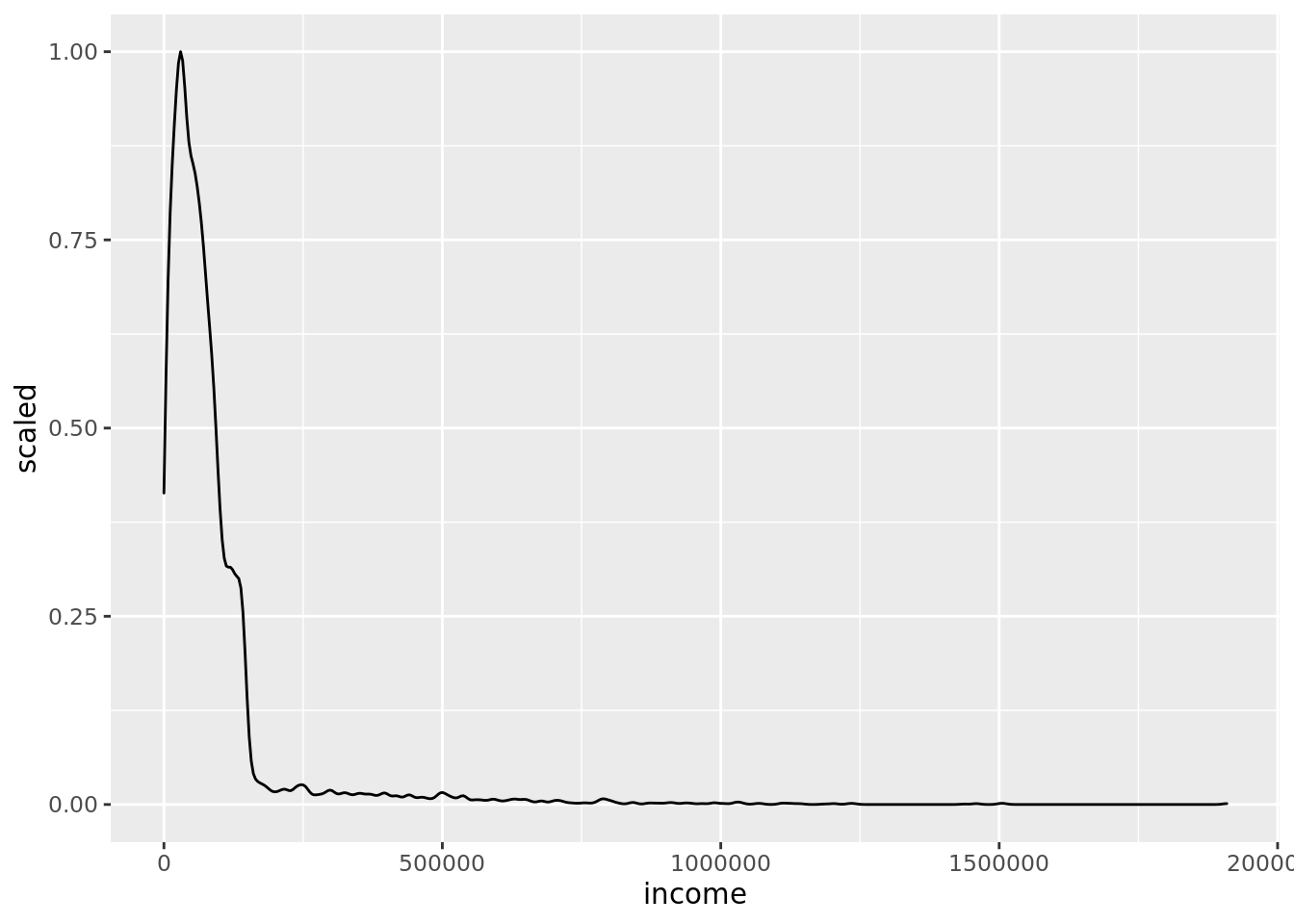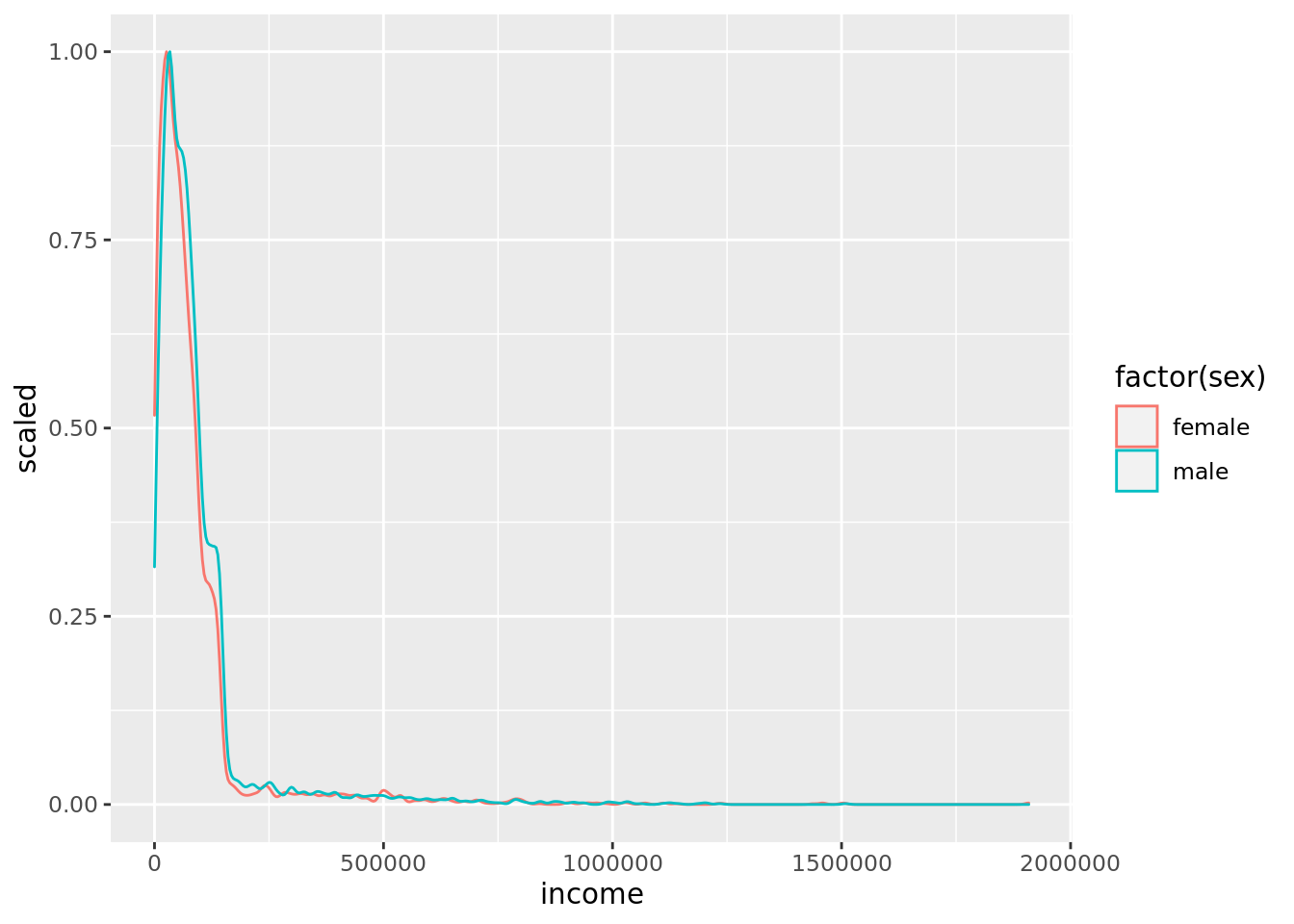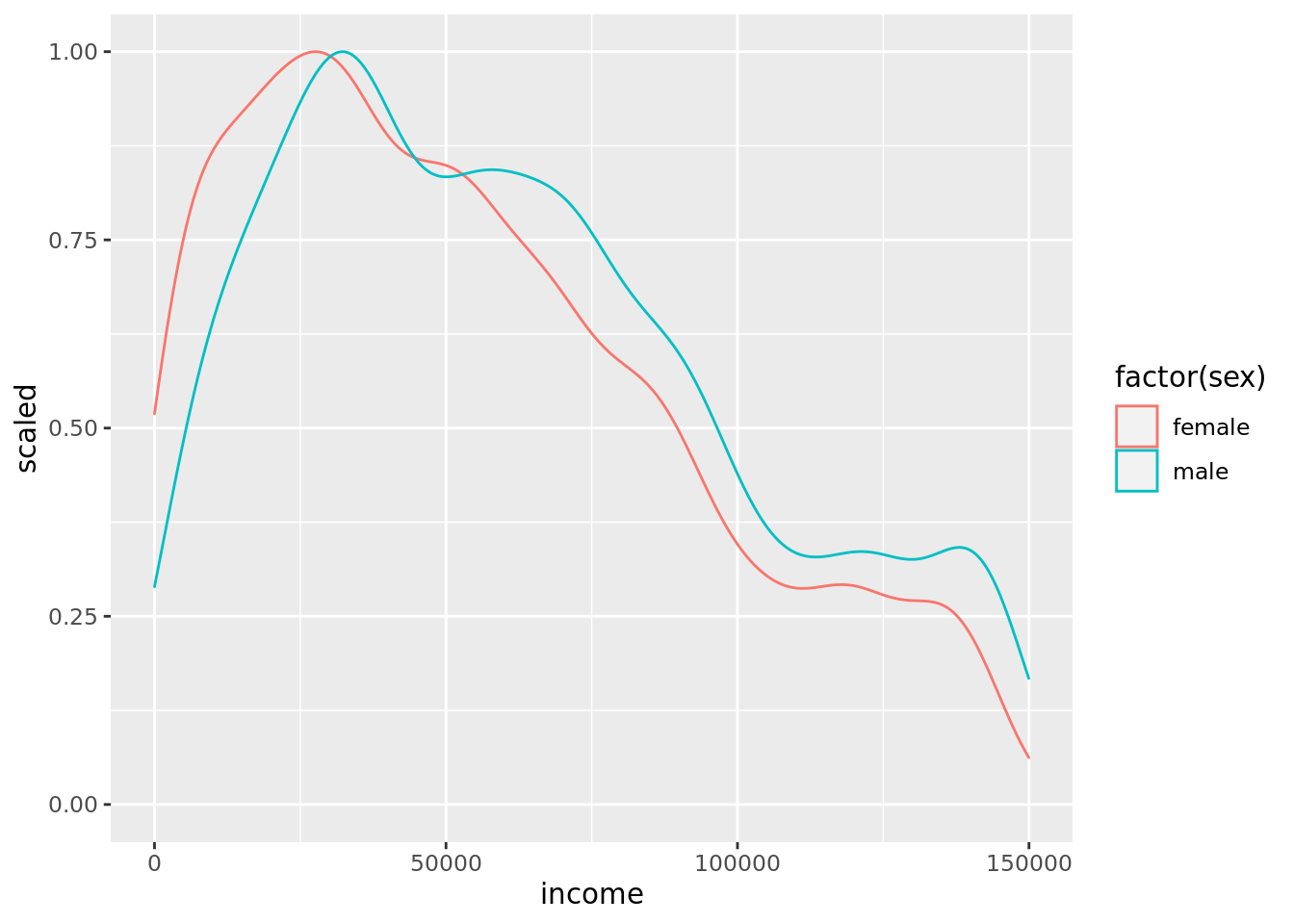## Before you start…

Before starting this exercise, you should have had a brief introduction to using RStudio – Introduction to RStudio. You should also have also completed the workshop exercises for Exploring Data. If not, take a look these earlier worksheets before continuing.

The first few steps are the same as in the Exploring Incomes workshop. First, log in to RStudio server, and make sure you are in your `psyc411` project.

Next, create a new script file in your project, called `groupdiff.R`.

Now, you load tidyverse and you load the income data frame, by adding the following commands to your R script, and using CTRL+ENTER to run each command in turn:

``library(tidyverse)``
``cpsdata <- read_csv(url("http://www.willslab.org.uk/cps2.csv"))``

As a reminder, here’s what each of the columns in the income data frame contain:

Column Description Values
ID Unique anonymous participant number 1-10,000
sex Biological sex of participant male, female
native Participant born in the US? foreign, native
blind Participant blind? yes, no
hours Number of hours worked per week a number
job Type of job held by participant: charity, nopay, private, public
income Annual income in dollars a number
education Highest qualification obtained grade-school, high-school, bachelor, master, doctor

## Gender pay gap

One of the most widely discussed issues concerning income is the difference between what men and women, on average, get paid. Let’s have a look at that difference in our teaching sample of 10,000 US participants.

We’ll start by calculating median income, irrespective of biological sex. You already did this in the Exploring data workshop, so this is revision. If you need to, take a look back at that last worksheet to remind yourself how this works:

``cpsdata %>% summarise(median(income))``

### Grouping data

What we need, though, are two median incomes – one for males and one for females. In R, the command `group_by` allows us to do this. In this case, we want to group the data by biological sex, so the command is `group_by(sex)`. We pipe (`%>%`) the data in `cpsdata` to the `group_by` command in order to group it, and then we pipe (`%>%`) it to `summarise` to get a summary for each group (a median, in this case).

So, the full command to add to your script and run (CTRL+ENTER) is:

``cpsdata %>% group_by(sex) %>% summarise(median(income))``
``````# A tibble: 2 x 2
sex    `median(income)`
<chr>             <dbl>
1 female           52558.
2 male             61746.``````

Looking at your output, you’ll be able to see that median income for males is around \$62,000, while for females it’s around \$53,000. (If you’re curious what the rest of the output means, including the word “tibble”, see more on tibbles). The message concerning “ungrouping output” just means that after R gives you the means, it forgets that you grouped the data (so if you want to use these groups again, you’ll have to ask for them again, see later).

R also works as a calculator, so we can work out female median income as a percentage of male median income – this is a standard way of expressing the gender pay gap:

``52558 * 100 / 61746``
`` 85.11968``

In conclusion, women in our made-up sample get paid, on average, around 85% what men get paid. This is about the same percentage as we see in the analyses of the gender pay gap in the US, using real data sets.

## Variation in pay

Of course, not every male gets \$62k a year in the US, and not every female gets \$53k. It seems very likely that the range of incomes earned by men and women overlap – meaning that if you picked one man and one woman at random, there’s a reasonable chance that the woman earns more than the man. This variation in pay is the topic of the next exercise.

Standard deviation is a number that basically represents how far, on average, people are from the mean. If the standard deviation of income is large, it’s quite likely that a person, picked at random, will have an income that’s a lot different to the mean. You can see this in the first few lines of our `cpsdata` data set – the participant with ID 5 has an annual income of over \$700k; more than 12 times the median income!

### Calculating standard deviation

What is the standard deviation in pay for males, and for females? We can calculate this in R with a minor change to the commands we’ve previously used. Specifically, we want a by-group summary, not of `median` income, but of the standard deviation, `sd`, of income.

``cpsdata %>% group_by(sex) %>% summarise(sd(income))``
``````# A tibble: 2 x 2
sex    `sd(income)`
<chr>         <dbl>
1 female      130449.
2 male        127601.``````

The standard deviations for both men and women are very large (around \$130k) compared to the size of the average pay gap (around \$9k). This means there is very considerable overlap in salaries between these two groups. It’s quite hard to get an intuitive sense of the size of this overlap just from the standard deviations, but a graph can help to make things clearer.

### Drawing a density plot

In the Exploring data worksheet, we used R to produce a histogram of incomes. The first thing we’re going to do now is to produce a scaled density plot of incomes. Scaled density plots can be interpreted much the same way as a histogram - the higher the curve is at a particular income, the more people who have that income.

The main difference between a scaled density plot and a histogram is that the highest point on a scaled density plot is always one. This can make it easier to compare two groups, particularly if one group has fewer people in it than the other.

So here’s the command to add to your script, that will do a scaled density plot for incomes, ignoring biological sex. It works the same way as the histogram command from last time, except that we’ve replaced `geom_histogram` with `+ geom_density`.

``cpsdata %>% ggplot(aes(income)) + geom_density(aes(y=..scaled..)) ``The part of the command `aes(y=..scaled..))` says that the aesthetic (‘aes’) we want for the y-axis of the graph is “scaled” … in other words we want a scaled density plot.

### Drawing a density plot for each group

Next, we’re going to produce two density plots, on the same axes – one for males, and one for females. This is so we can look at how much the two sets of incomes overlap.

We want these two density plots to be different colours, to make it easy for us to tell them apart. We do this by adding `colour=factor(sex)` to the aesthetics (`aes`) of the plot.

``cpsdata %>% ggplot(aes(income, colour=factor(sex))) + geom_density(aes(y=..scaled..)) ``Oh dear! That was not very revealing! These two lines seem basically on top of each other … but they can’t be because we know the two groups differ in median income by several thousand dollars. We have a problem to solve…

## Dealing with extreme data points

The problem is one of scale. As we discussed in Exploring data, there are a small number of people who earn very high salaries. In fact, both the highest-paid man, and the highest-paid woman in our sample earn considerably more than \$1m. We can find their exact salaries using the `max` (short for “maximum”) summary; here’s the command to add to your script and run:

``cpsdata %>% group_by(sex) %>% summarise(max(income))``
``````# A tibble: 2 x 2
sex    `max(income)`
<chr>          <dbl>
1 female       1908742
2 male         1508971``````

These figures relate to another big issue in the US (and many other countries) – income inequality. When we work out income inequality for a company (e.g. Starbucks), we take the salary of the CEO and divide it by the median salary of the workers. The CEO of Starbucks earns about 700 times as much as the average worker at Starbucks. In our sample of 10,000 people, the best paid woman earns about 36 times more than the average (median) woman:

``1908742 / 52558``
`` 36.31687``

### Filtering data

Somehow, we need to deal with the fact that a few people in our sample are very well paid, which makes the difference between men and women hard to see on our graph, despite the difference being in the range of several thousand dollars a year. One of the easiest ways around this is to exclude these very high salaries from our graph.

Looking at our previous density plots, we can see that the vast majority of people are paid less than \$150k a year. So, let’s restrict our plotting to just those people.

We do this using the `filter` command. It’s called filter because it works a bit like the filter paper in a chemistry lab (or in your coffee machine) – stopping some things, while letting other things pass through. We can filter our data by telling R what data we want to keep. Here, we want to keep all people who earn less than £150k, and filter out the rest. So the filter we need is `filter(income < 150000)`, where `<` means “less than”.

We’ll be using this dataset of people with <\$150k incomes a few times, so we’re going to give it a new name, `cpslow` (or any other name you want, e.g. angelface )

So, what we need to do is pipe (`%>%`) our `cpsdata` data to our `filter(income < 150000)`, and use an arrow, `<-`, to send this data to our new data frame, `cpslow`. Recall that `<-` sends the thing on its right to the thing on its left, so the full command to add to your script and run is:

``cpslow <- cpsdata %>% filter(income < 150000)``

We can take a look at this new data frame by clicking on it in RStudio’s Environment window. By looking at the ID numbers, you can see that some people in our original sample have been taken out, because they earned at least \$150k.

Now, we can plot these filtered data in the same way as before, by changing the name of the dataframe from `cpsdata` to `cpslow`.

So start with the command `cpsdata %>% ggplot(aes(income, colour=factor(sex))) + geom_density(aes(y=..scaled..))`, make that change, add it to your script, and run it..

If you’ve got it right, your graph will look like this:At first glance, the two distributions of incomes still look quite similar. For example, the modal income – the point where the graph is highest – is at quite a low income, and that income is quite similar for both men and women. However, on closer inspection, you’ll also see that the red line (females) is above the blue line (men) until about \$60k, and below the blue line from then on. This means that more women than men earn less than \$60k, and more men than women earn more than \$60k.

So, the gender pay gap is visible in this graph. The graph also illustrates that the difference in this sample is small, relative to the range of incomes.

Note: This doesn’t mean that the gender pay gap is less (or more) important than income inequality. These kinds of questions of importance are moral, philosophical, and political. Statistics cannot directly answer these kinds of questions, but they can provide information to inform the debate.

## Introducing effect size

Effect size is a way of talking about the size of the difference between group means, relative to the standard deviations of those groups. We often use the letter d to stand for effect size.

If a difference has an effect size of 1, the difference in means is equal to the standard deviation. In social science, an effect size of 1 is considered “large” – in other words, many of the things we are interested in have an effect size smaller than 1.

At the other end of the scale, an effect size of 0.2 is considered to be “small”. Note that “small” refers to the size of the effect relative to the standard deviation, not its importance to society. If you have an effect size of 0.2, the difference between your groups is one-fifth the size of the standard deviation.

Below are some examples of small, medium, and large effect sizes. In these examples, each group has 100 participants.